## 5.2 Deviations & Scalars; Directionals & Vectors

### A. A Review of the Lower Derivatives

#### Taking stock or Where we've been and where we are going

In the preceding discussion we discovered how to generate the primary derivative of a Data Stream, the Decaying Average. This was not just one Decaying Average to characterize this growing set. Instead we generated a Data Stream of Decaying Averages. With each new Data entry, there is a new Decaying Average generated. This stream of Decaying Averages is a derivative of the Raw Data Stream. It is based upon averages.

#### Decaying Average = the Primary Derivative

In this section we are going to examine two different types of derivative sets, which are both outgrowths of the stream of Decaying Averages. Because of the primacy of the Decaying Average Stream, in generating these two unique derivatives, we will call it the Primary Derivative.

#### The First Deviation and the 'Scalar' Change Stream

The first of these derivatives we have already seen in the previous notebook. It is based upon the magnitude of changes. It was calculated to determine the Realm of Probability. In traditional statistics designed to describe Static Data Sets, the figure is called the Standard Deviation. We derived a Decaying Standard Deviation to characterize these Living Data Streams. We saw that the Standard Deviation with its square roots was more than we needed to describe the predictably unpredictable Living Stream. All we needed was the Decaying 'Average Deviation', which was generated in a similar way to the Decaying Averages. In fact it represents the Decaying Average of the 'Scalar' Change Stream, which we will explore below. We will call it the First Deviation.

#### Derivative Data Streams

In summing up our state of understanding, we have the Living Data Stream of Raw Data. We also have a Data Stream of Decaying Averages derived from the Raw Data, designed to generate a relevant and sensitive Running Average. This is not a Living Data Stream; it is a Derivative Data Stream. Finally we also have a Data Stream of Average Deviations, derived from the Decaying Averages Stream. This is also a Derivative Data Stream. The Raw Living Data Stream is the only one that is truly Alive. All the rest are derivatives. Similarly in physical dynamics: the only true Data Stream is that of position; the rest, i.e. velocity, acceleration, etc are all derivative.

#### The Scalar Change Stream or the First Scalar and the 2nd Deviation

Both the Average Stream and the Deviation Stream are averages. The Average Stream is derived from the Raw Data Stream. The Deviation Stream is derived from the scalar difference between the Present Data and the Past Average. This stream of absolute differences, we will call the Scalar Change Stream or the First Scalar. This is the Change Stream that the Deviation stream is the Average of. Below is its algebraic representation.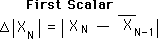### B. Terminology of Data Streams

#### No need for the term 'Stream'

Before going on let us use the above equation to explore and simplify Data Stream terminology. First each element in the above equation represents a Data Stream. The elements of a Data Stream are identified by their subscripts. If it doesn't have subscripts, it is not a Data Stream. Thus after we have named all of our elements, we will leave off their last name, the word 'Stream', unless desired for clarity or emphasis. Thus we can talk about the Decaying Average with the understanding that it is a Data Stream without having to specify it as such.

#### Data is the Raw Data Stream

The XN above represents the Raw Data Stream. There is only one Raw Data Stream. It could be Live Data, Dead Data, or Random Data. But there is only one Raw Data Stream from which all the rest are derived. Thus we can call this simply the Data. In these Notebooks, any time a 'capitalized' Data is found it refers to a Raw Data Stream.

#### Capitalized terms refer to Data Stream measures

We will be using many common terms to describe our Data Streams, for instance Stream, Average, Deviation, Vector, Scalar and Data. When these are capitalized they refer to the Data Stream measures, defined, described, and analyzed in these pages.

#### All the rest are Derivative Data Streams

All the Streams, except the Raw Data Stream, are called Derivative Data Streams. We don't need to refer to this aspect of their nature ever again, except for emphasis.

#### The Decaying Average Stream is the Average

The Decaying Average Derivative Data Stream is the Primary Derivative from which the others are derived. We will refer to this as the Decaying Average Stream, the Decaying Average, the Average Stream, or simply the Average. Note the capitalizations.

#### The Deviation and the Directional

We have already introduced the Decaying Average Deviation Data Stream and the Decaying Average Directional Data Stream in the Decaying Average Notebook. We will refer to the first as the Average Deviation, the First Deviation, (we'll see why soon) or simply the Deviation. We will refer to the second as the Directional Stream, the First Directional, or simply the Directional.

#### N = Present, N-1 = Past

Including our sample equation, we will have many equations with both the N and N-1 in the same equation. The N of XN represents the Nth Data point in the Data Stream. The N-1 represents the Data point before it in the ordered sequence. Thus when we see the N as a subscript, we will refer to it as the Present or the Now. When we see N-1 as a subscript we will refer to it as the Past. For instance, in the above equation, we could restate it verbally in this fashion. 'The Present Scalar is equal to the absolute difference between the Present Data and the Past Average.'

#### Present assumed when there is no adjective

When there is no adjective preceding a Data Stream measure, we will assume 'Present'. Thus we could restate the equation in this way. 'The First Scalar is equal to the absolute difference between the Data and the Past Average.'

#### The Scalar and the Vector

Just as the Deviation refers to the First Deviation and the Directional refers to the First Directional, so also does the Scalar refer to the First Scalar. Also when we come to our the last Data Stream measure, the Vector Data Stream, defined and described shortly, we can also refer to the First Vector as the Vector. So at last we can restate the equation in its most simplified version. 'The Scalar is equal to the absolute difference between the Data and the Past Average.' Now that we have simplified our terminology we can move back to our analysis of these many Data Streams.

### C. The Higher Derivatives and their Sets

#### The Second Scalar and Second Deviation

In review, from our Raw Data we generated the Average Stream. From the absolute difference between our Data and the Past Average we generated the Scalar Stream or First Scalar. Averaging this Stream we got the Deviation Stream. Similarly we could now generate a Second Scalar Stream based upon the difference between the First Scalar and the Past Deviation. This is represented below.This called the Second Scalar Change Stream or the Second Scalar. From this Change Stream we can generate a new Decaying Average Stream. This is called the Second Decaying 'Average Deviation' 'Derivative Stream' or more simply the Second Deviation. It is shown below.#### The Third Change Stream and the Third Deviation

Similarly we could generate a Third Scalar Change Stream based upon the difference between the Second Scalar and the past Second Deviation. This is shown below.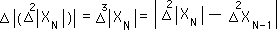We could then generate an Average Stream based upon this Change Stream. This is the Third Deviation and shown below.#### General Equations for Deviations and Scalars

The pattern should be apparent by now. First a Change Stream, then an Average of this stream which generates another Change Stream which then generates another Average Stream. This process could be repeatedly indefinitely. The general equation for the Change stream would be as follows.In words this equation roughly says that the P th Scalar is the difference between the Present (P-1) Scalar and Past (P-1) Deviation. From this Change Stream we generate the general equation for the Deviation Stream below.In words this equation roughly says that the Present Pth Deviation is equal to the sum of the Pth Scalar and the past Pth Deviation, both appropriately scaled. {We will discuss 'scaling' in another Notebook.}

#### The Scalar Series and the Deviation Series

We will gather all of these Scalar Streams into a set and call it the Scalar Series, because it is a series of Scalar Streams. This is shown below.Further we will gather all the Deviation Streams into a set and call it the Deviation Series, because it is a series of Deviations. Duh.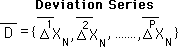#### Directionals are averages based upon Vector Streams

We can perform the exact same processes with Directionals. Remember from the Decaying Averages Notebook that Directionals are the Decaying Average of the difference between the Raw Data Stream and the Past Average. The only difference between Deviations and Directionals is that the Deviations are based upon scalar differences while the Directionals are based on differences that reflect both magnitude and direction. In our discussion, we have only talked about positive and negative directions. However our Directionals and there Change Streams could easily be treated as vectors of any dimension, using the same type of averaging equations. Hence our Deviations are scalars while our Directionals are actually vectors, with directions and magnitudes, which can be averaged in the Decaying fashion that we have outlined above. Thus we will call the Change Streams that the Directionals are based on Vector Change Streams or Vector Streams.

#### General Equations for the Directionals and their Change Streams

The Directionals are the Decaying Average Streams of the Vector Change Streams. The Vector Change Streams are represented generally as:In words this equation roughly says that the Pth Vector Stream is the difference between the Present (P-1) Vector Stream and the Past (P-1) Directional. From this Change Stream we generate the general equation for the Directional Stream below.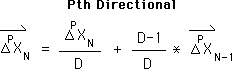In words this equation roughly says that the Pth Directional is equal to the sum of the Present Pth Vector and the past Pth Directional, both appropriately scaled.

#### The Directional Series and the Vector Series

All the Vector Streams are put into a set called, appropriately, the Vector Series. Its general representation is shown below.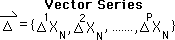Further we will gather all the Directional Streams into a set and call it the Directional Series, because it is a series of Directionals. The general representation is shown below.Because the Directionals and the Deviations are based upon averages their series are called Average Series. Because our Vector Streams and Scalar Streams are based upon differences or changes their series are called Change Series.

### D. Reflections upon the Connections between Raw Data, their Derivatives, and the Real World

#### Contextual, self-referential equations

As an aside, because our equations are contextual rather than content based, the elements are always functions of each other rather than functions of something else. They are self-referential equations. We will be examining this feature in depth in future Notebooks.

#### Four types of Derivative Streams

In summary, we have the Raw Data Stream from which an infinite number of Data Streams are derived. (As an aside this Data Stream could be Live, Dead, or Random; but the Data must be generated independent of the rest of the streams.) Appropriately these derivative Data Streams are also called Data Stream Derivatives. From the Raw Data Stream an Average Stream is derived. This is called the Primary Derivative or Decaying Average. From this Average Stream, two types of Average Streams and two types of Change Streams are derived. One of the types of Change Streams, the Scalar Stream, is based upon the absolute magnitude of differences. The Averages of these Streams are called Deviation Streams. The other type of Change Stream is based upon the magnitude and direction of differences and is called a Vector Stream. The Averages of these Streams are called Directional Streams. Further we gathered each type of Derivative Stream into their own sets, two average and two change sets.

#### The Reality of the Real Data

Let us not lose contact with the Reality of the Real, not derived, Data. The Data generates a Running Average, which has a Deviation and Directional. The Deviations set the Realm of Probability for the Live Data Stream, while the Directionals set the direction or tendencies of our Stream. The Change Series, by themselves mean very little to the organism. They are used to generate the Average Series and then thrown away. Their values are numbers that are crunched into the Average Series, which we will see in future notebooks are extremely relevant to the survival of the organism or business.

#### Computational Tools invested with momentum and emotional content

In these studies we will see that these three computational tools, which provide information that is extremely relevant to survival, also are invested with the momentum of habit, for good or ill. Further these same 3 computational tools, which have been so important evolutionarily, are also invested with a lot of emotional content. Because their influence is so fundamental as the link between survival, habit, and emotion, the study of their dynamics will prove exceedingly fruitful. But first let us look at some of their properties.